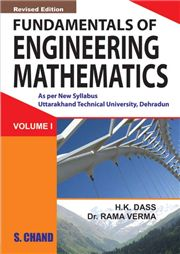# Fundamentals of Engineering Mathematics Vol-I (Uttrakhand), 4/e H K Dass & Rama Verma-S.CHAND

350.00 315.00

Useful Results •Unit – I: Matrices •Algebra Of Matrices •Rank Of Matrix •Solution Of Simultaneous Equations •Eigen Values & Eigen Vectors •Unit Ii: Differential Calculus – I •Ordinary Differentiation And Leibnitz’S Theorem •Unit Iii : Differential Calculus – Ii •Partial Differentiation •Total Differentiation •Expansion Of Function Of Several Variables •Jacobians •Errors •Extrema Of Function Of Several Variables •Curve Tracing •Unit – Iv : Multiple Integrals •Double Integrals •Triple Integral •Gamma, Beta Functions, Differentiation Under The Integral Sign •Unit – V : Vector Calculus •Vector Differentiation •Vector Integration •Question Papers •Index